# Kirchhoff’s First Law

Kirchhoff’s laws are fundamental to circuit theory. They quantify how current flows through a circuit and how voltage varies around a loop in a circuit. Kirchhoff’s circuit laws were first described in 1845 by the German physicist Gustav Kirchhoff.

## Kirchhoff’s Junction Rule

Kirchhoff’s first law goes by several names as Kirchhoff’s Current Law (KCL), Kirchhoff’s Junction Rule, Kirchhoff’s point rule, Kirchhoff’s nodal rule. It is an application of the principle of conservation of electric charge. The law states that at any circuit junction, the sum of the currents flowing into and out of that junction are equal.

In simple terms, what KCL really says is that,

The sum of all currents entering a node is equal to the sum of all currents leaving the node.

We perform analysis on all nodes based on the inflow and outflow of current. Current directions at the node are based on presumed directions of the currents. As long as the assumed directions of the currents are consistent from node to node, the final result of the analysis will reflect the actual current directions in the circuit.

Mathematically, Kirchhoff’s Current law is stated as follows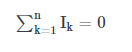where n is the total number of branches carrying current towards or away from the node.

Since you are here, you might be interested in the following articles:

## Solving Circuits Using KCL

Let us look at a few examples that demonstrate the law in practice and understand its importance in determining the unknown parameters.

Example 1: Let us consider a network of assumed directions of the current as shown below.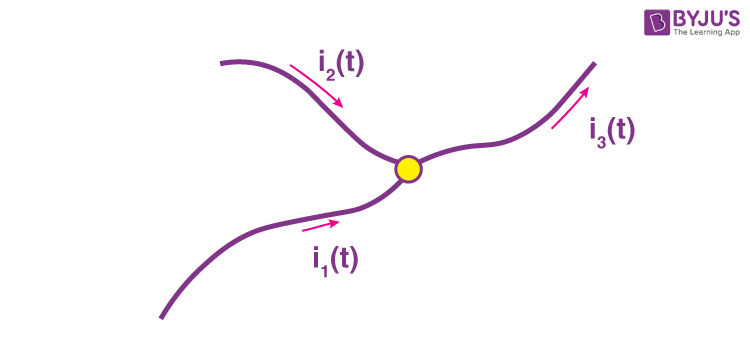Let us choose a sign convention such that currents entering the node are positive, and the currents leaving the node are negative. With this convention, KCL applied at the node yields the equation:

$$\begin{array}{l}i_{1}(t)+i_{2}(t)-i_{3}(t)=0 \,\, \textup{or} \,\, i_{1}(t)+i_{2}(t)=i_{3}(t)\end{array}$$

This amounts to the statement that the total current entering the node is the same as the total current leaving the node.

Example 2: Using KCL to determine the value of the unknown current i in the circuit below.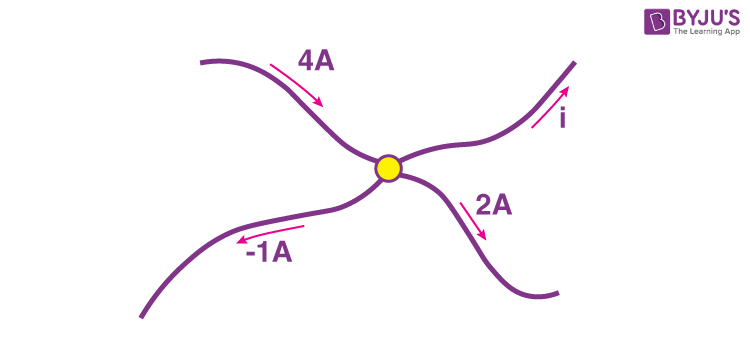Assuming that the current entering the node is positive, the sum of the currents is given by the equation,

$$\begin{array}{l}4A-(-1A)-2A-i=0\end{array}$$
$$\begin{array}{l}\Rightarrow 4A + 1A -2A = 3A\end{array}$$

Therefore, i = 3A, leaving the node(since our assumed direction of i on the diagram is that it is leaving the node, and our numerical value for i is positive).

### Disadvantages of KCL

• KCL is valid only if the total electric charge is constant in the circuit.
• KCL is suitable for high-frequency AC circuits.

Together Kirchhoff’s Voltage Law and the Current Law are a great pair of tools useful in analyzing electric circuits.

## Practice Questions

1. Calculate currents I_{1} and I_{2} in the given circuit?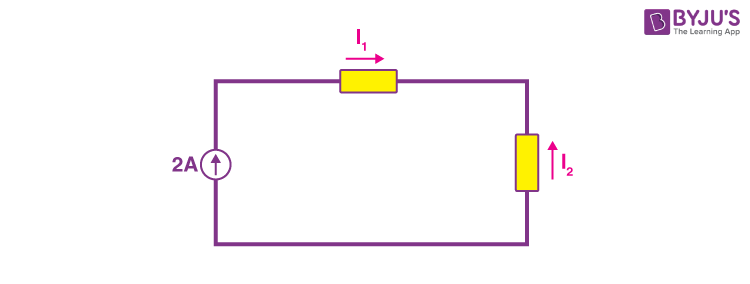Solution:

Let us identify the different nodes in the circuit as A and B.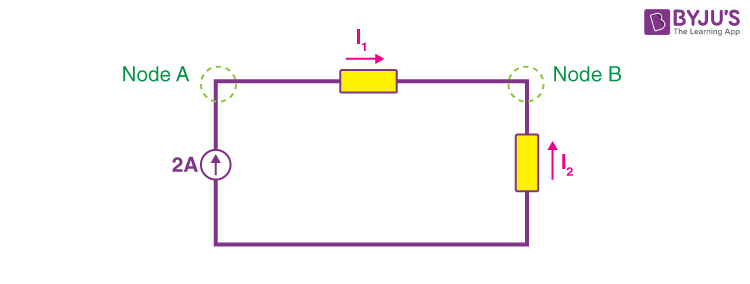If the current entering the nodes are assumed positive, then 2 A – I1 = 0. Therefore, I1 = 2 A.

Applying KCL at node B, we get the equation I1 + I2 = 0. Since we know the value of I1, we can easily determine the value of I2 as –2 A.

2. Calculate the currents I1 and I2, in the circuit below.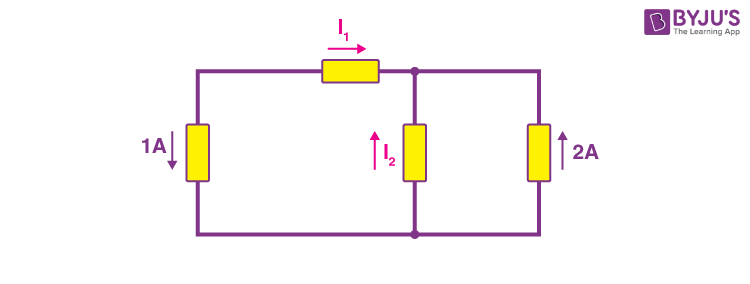Solution:

Let us identify the two nodes in the circuit as A and B.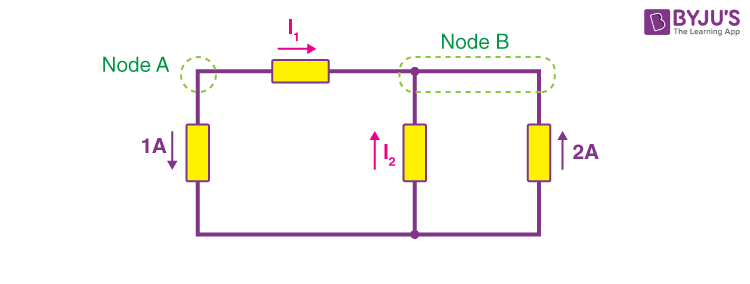Now applying KCL to node A, assuming the currents leaving the nodes as positive, we get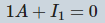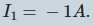Now applying KCL to node B, we get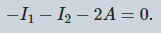Substituting the value of I1 in the above equation, we get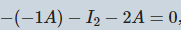Solving, we get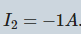## Watch the video and learn basic electrical circuit concepts and learn to solve problems on Electrical Circuit, KCL, and KVL.## Frequently Asked Questions – FAQs

### Who put forth Kirchhoff’s circuit laws?

Kirchhoff’s circuit laws were put forth by Gustav Kirchhoff, the German physicist.

### What is Kirchhoff’s first law also known as?

Kirchhoff’s first law is also known as Kirchhoff’s nodal rule, Kirchhoff’s Junction Rule, Kirchhoff’s Current Law (KCL), and Kirchhoff’s point rule.

### State Kirchhoff’s Current Law?

The sum of all currents entering a node is equal to the sum of all currents leaving the node.

### What are non-mechanical waves?

$$\begin{array}{l}\bigtriangledown . D = \rho _{v}\end{array}$$
$$\begin{array}{l}\bigtriangledown . B = 0\end{array}$$

### How is Kirchhoff’s Current law represented mathematically?

$$\begin{array}{l}\sum_{k=1}^{n} I_{k}=0\end{array}$$
Test your knowledge on Kirchhoff's first law